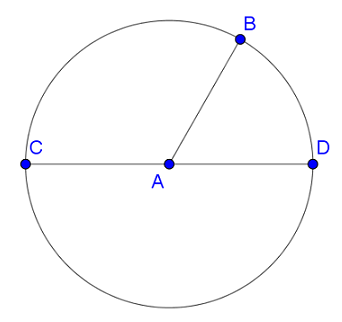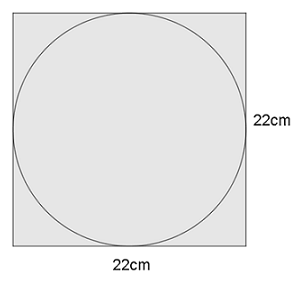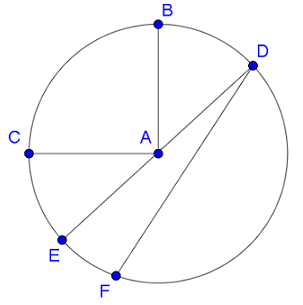# Geometry

## Objective

Define circle and identify the measurements radius, diameter, and circumference.

## Common Core Standards

### Core Standards

?

• 7.G.B.4 — Know the formulas for the area and circumference of a circle and use them to solve problems; give an informal derivation of the relationship between the circumference and area of a circle.

## Criteria for Success

?

1. Understand that a circle is a closed shape defined by the set of all points that are the same distance from the center point of the circle.
2. Understand that the radius is the distance or the line segment from the center of the circle to any point on the circle.
3. Understand that the diameter is the distance or the line segment from one point on the circle, through the center, to another point on the circle.
4. Understand that the circumference is the measurement of the distance around the circle.

## Tips for Teachers

?

The following materials are needed for this lesson: compasses, rulers, and blank paper.

#### Remote Learning Guidance

If you need to adapt or shorten this lesson for remote learning, we suggest prioritizing Anchor Problem 2 (benefits from worked example). Find more guidance on adapting our math curriculum for remote learning here.

#### Fishtank Plus

• Problem Set
• Student Handout Editor
• Vocabulary Package

## Anchor Problems

?

### Problem 1

Draw a circle in two ways:

Method 1:

• Draw a point on a blank piece of paper and label it $P$.
• Using a ruler, measure exactly 2 inches from point $P$ and make a point.
• Measure exactly 2 inches from point $P$ in a different direction and make a point.
• Continue measuring and adding points until the shape of a circle starts to appear.

Method 2:

• Draw a point on a blank piece of paper and label it $Q$.
• Using a compass, set the measurement to $1\frac{1}{2}$ inches.
• Draw a circle using the compass.

Based on your drawings, how would you define a circle?

### Problem 2

A circle is shown below with center point $A$, and points $B$, $C$, and $D$ on the circle.1. Use your ruler to find the measurements below:
1.  $\over AB$
2. $\over AC$
3. $\over AD$
4. $\over{CD}$
2. What is the relationship between the radius and diameter of a circle?
3. Draw another point, $E$, on the circle that could be used to create diameter $\over{BE}$.

### Problem 3

Hilary is ordering a large circular pizza from her local pizza shop. She asks about the size of the pizza and is given 3 different values: 14 inches, 44 inches, and 7 inches.

Hilary knows three measurements that are used to describe circles: radius, diameter, and circumference. Which value best matches each measurement? Explain your reasoning.

## Problem Set

?The following resources include problems and activities aligned to the objective of the lesson that can be used to create your own problem set.

• Include problems where students draw circles given measurements and appropriate tools.
• Include examples of noncircles, such as ovals, and ask students to explain why they are not circles and to justify their thinking.
• Include examples of nondiameters, such as line segments drawn across the circle that do not pass through the center, and ask students to explain why the line segment does not represent a diameter.
• Include images of a circle inscribed in a square with the side lengths of the square given, and ask students to identify the diameter and radius of the circle; for example:?

The circle below has a center at point $A$ and a radius of 4.5 cm.Which of the line segments below can you determine the measurement of? Find the measurement of the line segments that you can determine. For any one you cannot determine, explain your reasoning why.

a.  $\over AB$

b.    $\over AC$

c.    ${\over DF}$

d.    $\over AE$

e.   ${\over DE}$

f.    $\over AD$

?